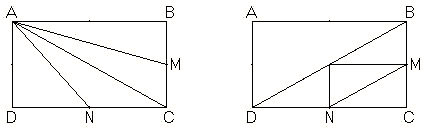# Geometry, Algebra, and Illustrations

A simple multiple choice problem with solution has been posted at the MAA site:

The area of a rectangle ABCD is 72. If point A and the midpoints of BC and CD are joined to form a triangle, the area of the triangle isThe problem came from the 2000 AMC 8 (#25). The solution given is purely algebraic.

### Solution

Three triangles lie outside ΔAMN. Their areas are 1/4, 1/4, and 1/8 for a total of 5/8 of the rectangle. The area of ΔAMN is 73·3/8 = 27.

Let the rectangle have sides of 2a and 2b so that 4ab = 72 and ab = 18. Three right triangle lie outside ΔAMN, and their areas are (2a)(b)/2, a(2b)/2, ab/2 for a total of ab·5/2 = 18·5/2 = 45. The area of ΔAMN is 72 - 45 = 27.Now, the problem is pretty simple, and it was probably the way it was supposed to be solved. However, an illustration would not be amiss:ΔABC whose area is half that of the rectangle is divided into two (ABM and AMC) by the median AM so that each of the smaller triangles has the area 1/4 of that of the rectangle, i.e., 72/4 = 18. Similarly, area(ΔADN) = Area(ΔANC) = 18.

Drawing the second diagonal BD and the midlines of ΔBCD, we see that area(ΔCMN) is 1/8 of that of the rectangle: area(ΔCMN) = 72/8 = 9.

The above solution tells us that

area(ΔAMN) = area(ABCD) - area(ΔABM) - area(ΔADN) - area(ΔCMN) = 72 - 18 - 18 - 9 = 27.

The diagram also suggests another solution:

area(ΔAMN) = area(ΔAMC) + area(ΔANC) - area(ΔCMN) = 18 + 18 - 9 = 27.

While the latter could have been easily derived algebraically, I see the advantage of presenting the diagrammatic solution as less linear and, perhaps, more open minded. One diagram illustrates two solutions at once and serves, if only a little, more educational value than the cited solution.

But is not this the purpose of posting a solution in the first place?!